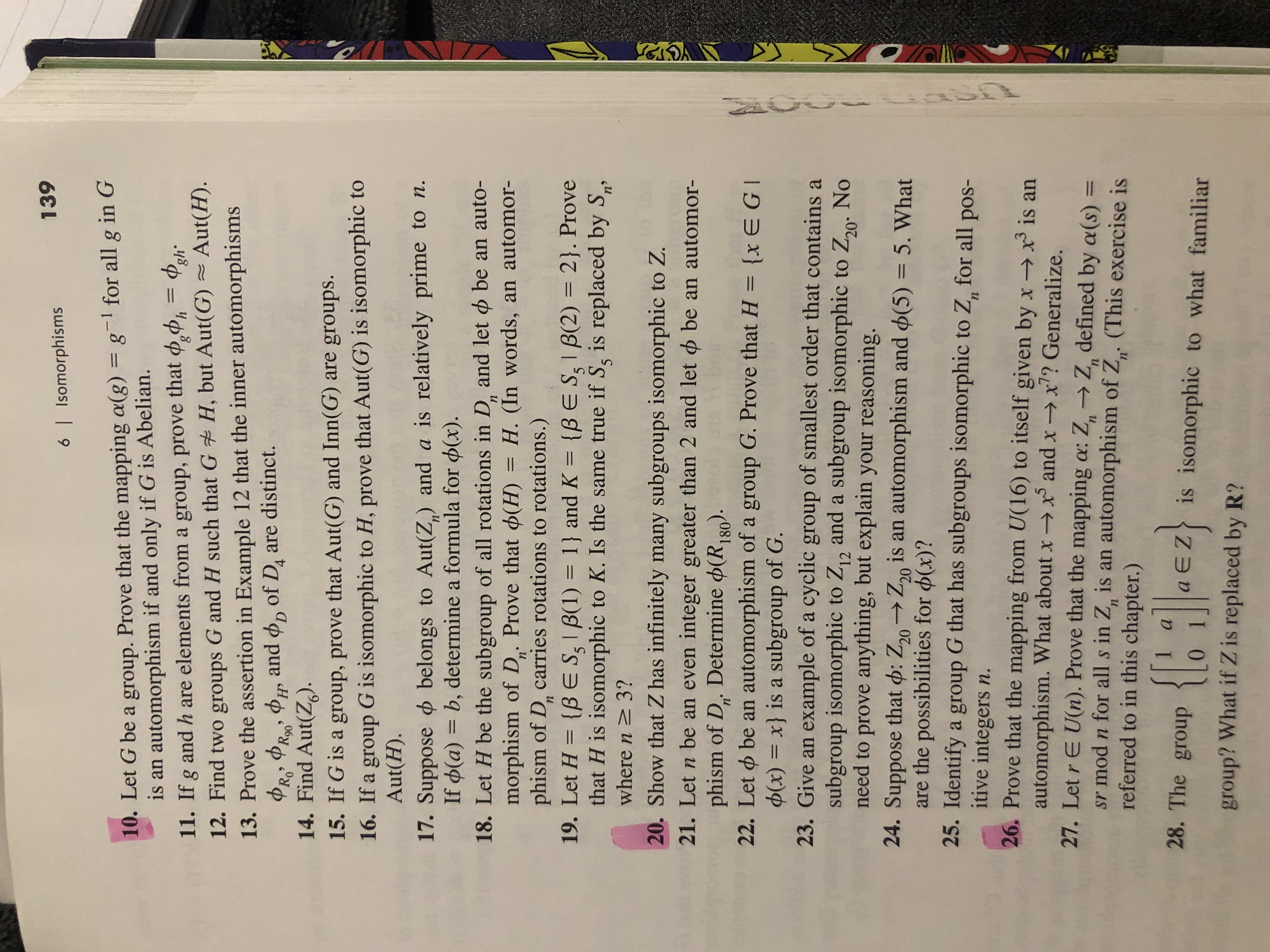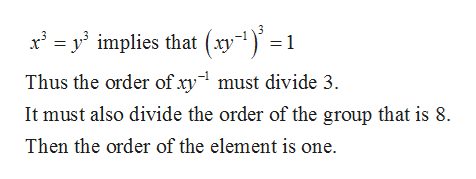# 1396 I Isomorphisms10. Let G be a group. Prove that the mapping a(g) = g1 for all g in Gis an automorphism if and only if G is Abelian.11. If g and h are elements from a group, prove that h12. Find two groups G and H such that G H, but Aut(G) Aut(H).13. Prove the assertion in Example 12 that the inner automorphismsand o, of Di are distinct.PRa14. Find Aut(Z)15. If G is a group, prove that Aut(G) and Inn(G)16. If a group G is isomorphic to H, prove that Aut(G) is isomorphic toAut(H).are groups.17. Suppose belongs to Aut(Z,) and a is relatively prime to n.If d(a) b, determine a formula for p(x).18. Let H be the subgroup of all rotations in D. and let d be an auto-morphism of D. Prove that (H) = H. (In words, an automor-phism of D, carries rotations to rotations.)19. Let H {BES,IB(1) 1} and Kthat H is isomorphic to K. Is the same true if S, is replaced by Swhere n 3?20. Show that Z has infinitely many subgroups isomorphic to Z.п11nn2}. Prove{BE SIB(2)п>21. Let n be an even integer greater than 2 and let o be an automor-phism of D. Determine (R, 80).22. Let be an automorphism of a group G. Prove that H {x EG(x) =x} is a subgroup of G23. Give an example of a cyclic group of smallest order that contains asubgroup isomorphic to Z12 and a subgroup isomorphic to Z20 Noneed to prove anything, but explain your reasoning.24. Suppose that d: Z20Z20 is an automorphism and (5) 5. Whatare the possibilities for d(x)?25. Identify a group G that has subgroups isomorphic to Z for all pos-itive integers n.26. Prove that the mapping from U(16) to itself given by x -x3 is anautomorphism. What about xx and x -x? Generalize.27. Let r E U(n). Prove that the mapping a: Z> Z defined by a(s) =sr mod n for all s in Z, is an automorphism of Z (This exercise isreferred to in this chapter.)n'28. The group aEZis isomorphic to what familiargroup? What if Z is replaced by R?U

Question
6 views

26.help_outlineImage Transcriptionclose139 6 I Isomorphisms 10. Let G be a group. Prove that the mapping a(g) = g1 for all g in G is an automorphism if and only if G is Abelian. 11. If g and h are elements from a group, prove that h 12. Find two groups G and H such that G H, but Aut(G) Aut(H). 13. Prove the assertion in Example 12 that the inner automorphisms and o, of Di are distinct. PRa 14. Find Aut(Z) 15. If G is a group, prove that Aut(G) and Inn(G) 16. If a group G is isomorphic to H, prove that Aut(G) is isomorphic to Aut(H). are groups. 17. Suppose belongs to Aut(Z,) and a is relatively prime to n. If d(a) b, determine a formula for p(x). 18. Let H be the subgroup of all rotations in D. and let d be an auto- morphism of D. Prove that (H) = H. (In words, an automor- phism of D, carries rotations to rotations.) 19. Let H {BES,IB(1) 1} and K that H is isomorphic to K. Is the same true if S, is replaced by S where n 3? 20. Show that Z has infinitely many subgroups isomorphic to Z. п 11 n n 2}. Prove {BE SIB(2) п> 21. Let n be an even integer greater than 2 and let o be an automor- phism of D. Determine (R, 80). 22. Let be an automorphism of a group G. Prove that H {x EG (x) =x} is a subgroup of G 23. Give an example of a cyclic group of smallest order that contains a subgroup isomorphic to Z12 and a subgroup isomorphic to Z20 No need to prove anything, but explain your reasoning. 24. Suppose that d: Z20Z20 is an automorphism and (5) 5. What are the possibilities for d(x)? 25. Identify a group G that has subgroups isomorphic to Z for all pos- itive integers n. 26. Prove that the mapping from U(16) to itself given by x -x3 is an automorphism. What about xx and x -x? Generalize. 27. Let r E U(n). Prove that the mapping a: Z> Z defined by a(s) = sr mod n for all s in Z, is an automorphism of Z (This exercise is referred to in this chapter.) n' 28. The group aEZis isomorphic to what familiar group? What if Z is replaced by R? U fullscreen
check_circle

Step 1

The group  U(16)={1,3,5,7,9,11,13,15}, the order of the group is 8 and it is abelian group.

Consider the mapping as,

Step 2

Let some x and y belongs to the group U(16), then

Step 3

Now consid...help_outlineImage Transcriptionclosexymplies that (xy"1)' =1 3 Thus the order of xy must divide 3 It must also divide the order of the group that is 8. Then the order of the element is one. fullscreen

### Want to see the full answer?

See Solution

#### Want to see this answer and more?

Solutions are written by subject experts who are available 24/7. Questions are typically answered within 1 hour.*

See Solution
*Response times may vary by subject and question.
Tagged in

### Math